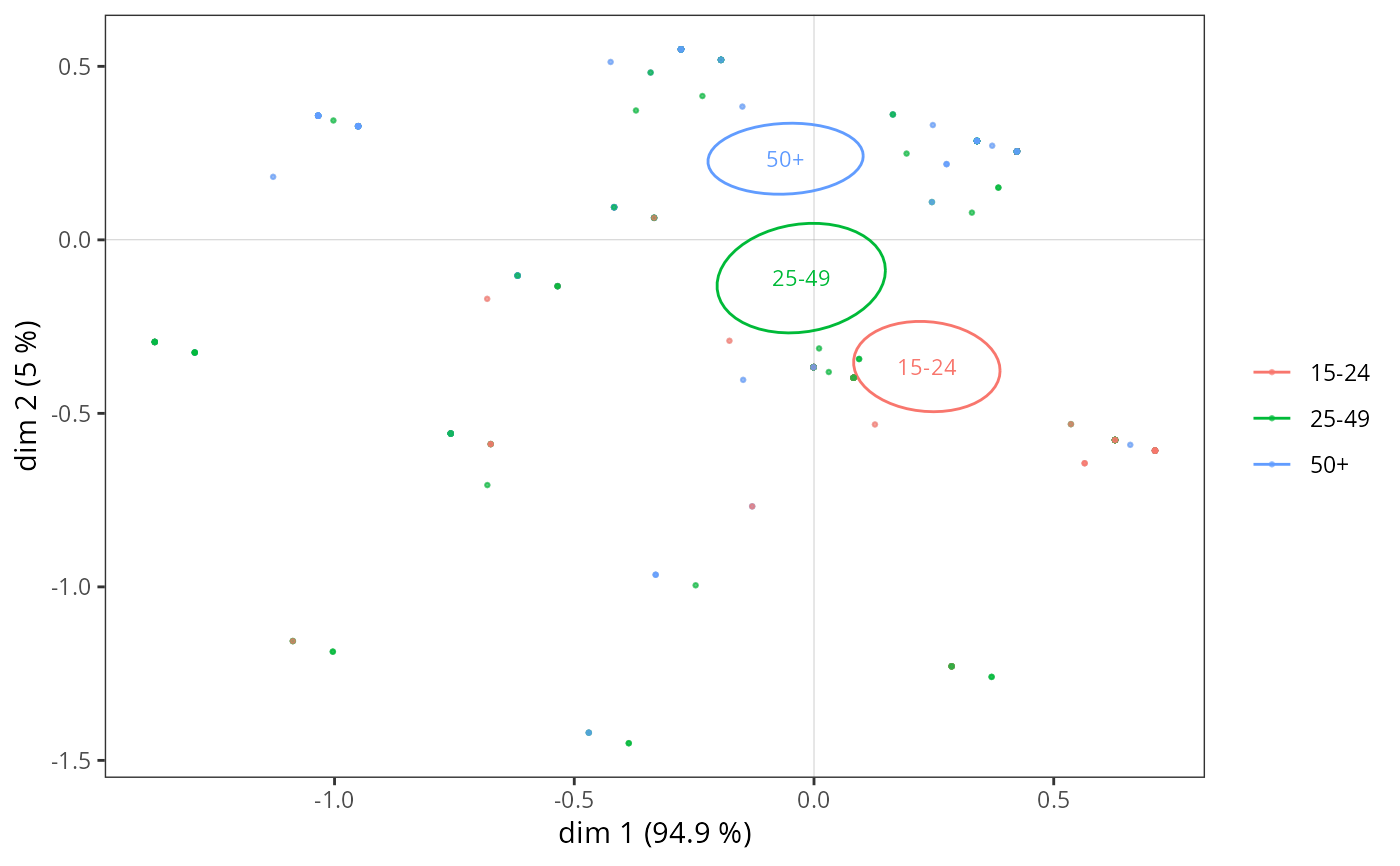Adds concentration ellipses and other kinds of k-inertia ellipses for a categorical variable to a MCA cloud of individuals.

ggadd_kellipses(p, resmca, var, sel = 1:nlevels(var), axes = c(1,2),
kappa = 2, label = TRUE, label.size = 3, size = 0.5, points = TRUE,
legend = "right")

## Arguments

p

ggplot2 object with the cloud of individuals

resmca

object of class MCA, speMCA, csMCA, stMCA or multiMCA

var

Factor. The categorical variable used to plot ellipses.

sel

numeric vector of indexes of the categories to plot (by default, ellipses are plotted for every categories)

axes

numeric vector of length 2, specifying the components (axes) to plot. Default is c(1,2).

kappa

numeric. The kappa value (i.e. "index") of the inertia ellipses. By default, kappa = 2, which means that concentration ellipses are plotted.

label

Logical. Should the labels of the categories be plotted at the center of ellipses ? Default is TRUE.

label.size

Size of the labels of the categories at the center of ellipses. Default is 3.

size

Size of the lines of the ellipses. Default is 0.5.

points

If TRUE (default), the points are coloured according to their subcloud.

legend

the position of legends ("none", "left", "right", "bottom", "top", or two-element numeric vector). Default is right.

## Value

a ggplot2 object

## Details

If kappa=2, ellipses are called "concentration" ellipses and, for a normally shaped subcloud, contain 86.47 percents of the points of the subcloud. If kappa=1, ellipses are "indicator" ellipses and contain 39.35 percents of the points of the subcloud. If kappa=1.177, ellipses are "median" ellipses and contain 50 percents of the points of the subcloud. This function has to be used after the cloud of individuals has been drawn.

## Note

Ellipses are colored according to the categories of the variable, using the default ggplot2 palette. The palette can be customized using any scale_color_* function, such as scale_color_brewer(), scale_color_grey() or scale_color_manual().

## Author

Nicolas Robette

ggcloud_indiv, ggadd_supvar, ggadd_supvars, ggadd_ellipses, ggadd_density, ggadd_interaction, ggsmoothed_supvar, ggadd_chulls, ggadd_corr

## Examples

# specific MCA of Music example data set
data(Music)
junk <- c("FrenchPop.NA", "Rap.NA", "Rock.NA", "Jazz.NA", "Classical.NA")
mca <- speMCA(Music[,1:5], excl = junk)
# concentration ellipses for Age
p <- ggcloud_indiv(mca, col = "lightgrey")